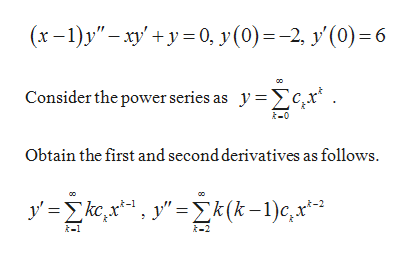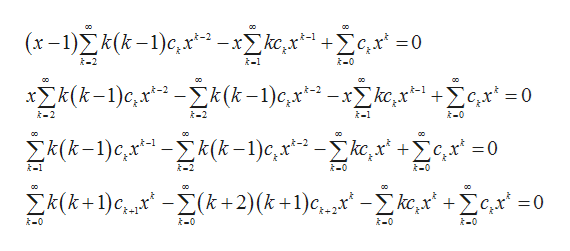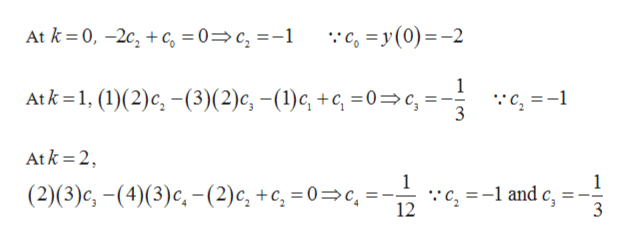# Differential EquationsSolve the initial value problem using the power series method:(x-1)y"-xy'+y=0,    y(0)=-2, y'(0)= 6

Question

Differential Equations

Solve the initial value problem using the power series method:

(x-1)y"-xy'+y=0,    y(0)=-2, y'(0)= 6

check_circleExpert Solution
Step 1

The differential equation is given as:help_outlineImage Transcriptionclose(x-1)y" 0, y (0)=-2 y'(0) 6 Consider the power series as ycx Obtain the first and second derivatives as follows. ykxy=2k(k-1)c,x*-2 fullscreen
Step 2

Substitute y and its derivatives in the differential equation and obtain the recurrence relation as follows.help_outlineImage Transcriptioncloseco Τ Τ ( x-)Σ (k- 1)ς,x*3 -Σkr,x* -1 Σc,x-0 k-2 -0 k-1 στ ΣΣk(k-1)c, πς -Σk(t-1)c,xt-3 xΣ k,x* +Σοχ =0 -2 -2 t-l k-0 Ε m Σ (k-1)ς,xα -Σ (k -1)c, x-Σk,x' +Σq -0 t-2 -0 t-0 Σ(k+ 1)c, Σ(k+2) (k + 1)c, ,-Σ&,χ' + Σς,χ' -0 t-0 -0 k-0 -0 fullscreen
Step 3

Substitute various values of k and o...help_outlineImage Transcriptionclose:с, 3у (0)—-2 At k %3D0, -2с, + с, %3D 0%3D с, %3-1 Atk%31, (1)(2)c, —(3)(2)с, -(1)с, +, %3D0%3D с, %3 :с, %3D-1 Atk 2 1 :с, %3-1 and c, 3 (2)(3)с, - (4)(3)с, - (2)с, +с, 3D0—с, 12 fullscreen

### Want to see the full answer?

See Solution

#### Want to see this answer and more?

Solutions are written by subject experts who are available 24/7. Questions are typically answered within 1 hour*

See Solution
*Response times may vary by subject and question
Tagged in

### Math